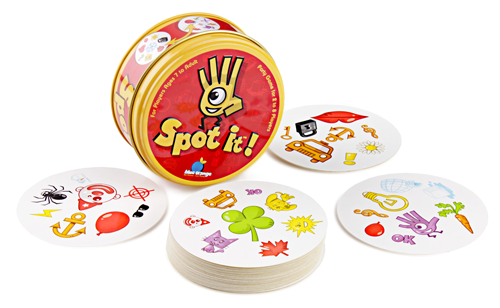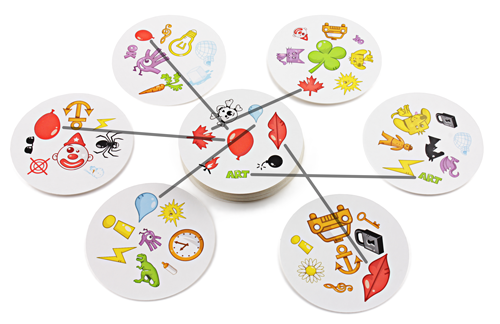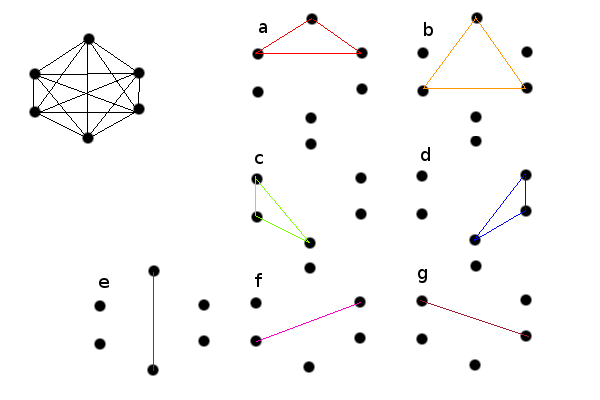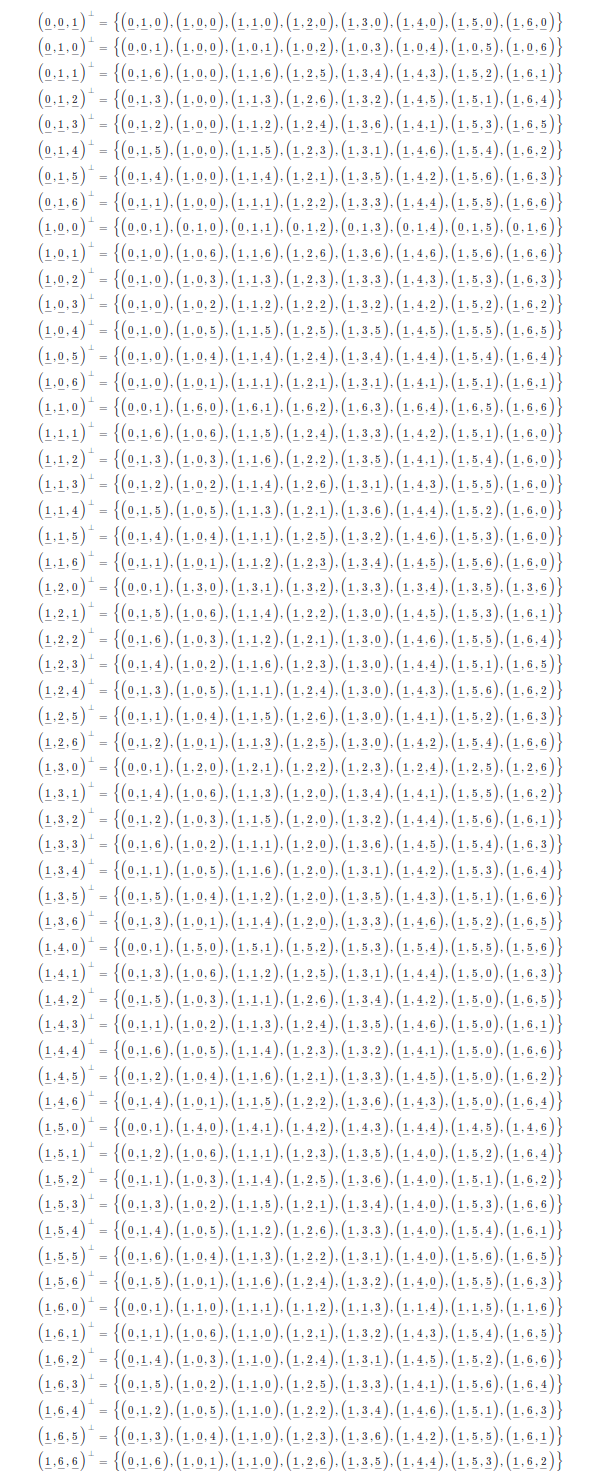# Card Games, Complete Subgraphs, Constant Weight Error Correcting Codes and Finite Projective Geometries

I was recently in a store buying a gift for a friend and found a card game that seemed interesting. The cards have small symbols which are arranged in a way such that between two cards there is always one symbol in common.These are the rules, from the store page:

Some people can spot a 90% off designer dress in a pile of discounted rubbish. They make out street signs from superhero distances. And they can identify the rarest of rare birds without even trying. You can be one of those people after a few quick rounds of Spot It! This smart game contains 55 illustrated cards with eight symbols each. There is always one, and only one, matching symbol between any two cards. All you need is a sharp eye and quick hands to take home the title of “Spot It! Master of the Visual Universe.” Win or lose, every player gets killer visual perception skills as a parting gift.

You can see an example of the relationship between cards here:As I was leaving the store I though maybe there is something more to this game than meets the eye.

## Card Games

First of all, how many symbols are there? And are they repeated or not? Let's start with the most simple approach. We have 55 cards, and we know that there is always a symbol in common between two cards. Given 55 cards we have a maximum of $$\left(\begin{array}{c} 55\\ 2\end{array}\right)=\frac{55\cdot54}{2}=1485$$ pairs of cards. Now, that seems a large number of symbols, more than one would imagine by looking at the card images. But the real problem with this approach is that each card would need 54 symbols, instead of just 8.

Let's think about it the other way around. We have 55 cards, with 8 symbols each, and each symbol is repeated at least twice. That makes $$\frac{55\cdot8}{2}=220$$ symbols. Obviously the problem here is that we can't guarantee that between any two cards there is always a symbol in common.

Now, if we think for a moment about the second question, what if the symbols were not repeated across more than 2 cards? Let $$C_{1}$$ be one card from the deck, it has 8 symbols, $$C_{1}=\left\{ s_{1},\ldots,s_{8}\right\}$$ which are shared by at most eight other cards $$C_{2},\ldots,C_{9}\,|\, C_{1}\cap C_{2}=\left\{ s_{1}\right\} \ldots C_{1}\cap C_{9}=\left\{ s_{8}\right\}$$ what happens when we consider yet another one, $$C_{10}$$. Then it has to be $$C_{1}\cap C_{10}\in\left\{ s_{1},\ldots,s_{8}\right\} \Rightarrow\exists i\in\left\{ 1,\ldots,8\right\} \,|\, C_{1}\cap C_{10}\cap C_{i}\neq\emptyset$$ Or in another words. With 8 symbols the card 1 could only be connected to 8 other cards, but not the remaining 46, arriving to a contradiction. As a matter of fact, if one looks carefully at the images of the deck and the video, it's possible to spot 4 cards that share the same symbol.

## Complete Subgraphs

The combinatorial approach didn't get me very far. Let's go back to the original problem and try to model it as a graph. We have 55 nodes, for each of the 55 cards. And each pair of nodes is connected through a symbol. So we have the complete graph of 55 nodes $$K_{55}$$, which has 1485 edges. Now we could think that each edge has a color, and that would reduce our problem, to the problem of finding a coloring of the dual graph of $$K_{55}$$. However that doesn't seem to work. In a coloring problem we are interested in assigning colors to nodes such that two asjacent nodes have different colors. In our case we can perfectly have two adjacent edges with the same color, corresponding to one card sharing a symbol with two other cards. In any case, the theory of graph coloring is very rich so there may be another less obvious approach that works.

Going back to our original graph $$K_{55}$$, realize that we can have edges coming from the same node that have the same color, for instance $$n_{1},n_{2},n_{3}\in V\left(K_{55}\right),\left(n_{1},n_{2}\right),\left(n_{1},n_{3}\right)\in E\left(K_{55}\right)$$ such that $$\left(n_{1},n_{2}\right),\left(n_{1},n_{3}\right)$$ have the same color. But this imposes the additional condition that $$\left(n_{2},n_{3}\right)$$ has the same color as the other two. Or in other words, taking all the edges that have the same color and the corresponding nodes should give us a complete subgraph. Going back to cards and symbols, this makes obvious sense. If we have 8 cards that share a particular symbol, then any two pairs of cards out of those 8 are connected through that same symbol. We know from the problem definition that there is no other symbol that can be shared among any pair of those 8 cards.

This seems closely related, but not quite the same as the problem of finding cliques in the graph. In our subgraphs each of the nodes is also connected to nodes outside of the subgraph. All other nodes, as a matter of fact. Nevertheless, considering that the clique cover problem is NP-complete, that doesn't augur an easily computable solution to our deck problem.

For the sake of example, let's try to find a solution in case we had 6 cards. We can start taking the complete subgraph with the first 5 nodes. All with the same color, or symbol a. And then the remaining node, with 5 edges to the previous 5. Each one with a different color b,c,d,e,f. We can't take the same color for all because the destination nodes are not connected within the subgraph. The 6 cards would be: $$\begin{eqnarray*} C_{1} & = & \left\{ a,b\right\} \\ C_{2} & = & \left\{ a,c\right\} \\ C_{3} & = & \left\{ a,d\right\} \\ C_{4} & = & \left\{ a,e\right\} \\ C_{5} & = & \left\{ a,f\right\} \\ C_{6} & = & \left\{ b,c,d,e,f\right\} \end{eqnarray*}$$ These verify that between each pair of cards there is only one symbol in common. However they don't satisfy the condition that each card have the same number of symbols. In order to satisfy that condition as well, we would need to make sure that each node is contained in the same number of subgraphs. With a little bit more work we can find a solution for $$K_{6}$$, which is as followsGiving the cards: $$\begin{eqnarray*} C_{1} & = & \left\{ a,b,e\right\} \\ C_{2} & = & \left\{ a,d,f\right\} \\ C_{3} & = & \left\{ b,d,g\right\} \\ C_{4} & = & \left\{ c,d,e\right\} \\ C_{5} & = & \left\{ b,c,f\right\} \\ C_{6} & = & \left\{ a,c,g\right\} \end{eqnarray*}$$ Again, it's easy to verify that between each pair of cards there is only one symbol in common. But now each card has only 3 symbols. In any case, following this approach it doesn't seem obvious how to manually scale the process to 55 cards.

## Constant Weight Error Correcting Codes

Here is another insight: we can write a matrix that has one row for each card, and one column for each symbol. This reminds to the incidence matrix for the graph, but is clearly different. The matrix is: $$\begin{array}{c} \begin{array}{ccccccc} a & b & c & d & e & f & g\end{array}\\ \left(\begin{array}{ccccccc} 1 & 1 & 0 & 0 & 1 & 0 & 0\\ 1 & 0 & 0 & 1 & 0 & 1 & 0\\ 0 & 1 & 0 & 1 & 0 & 0 & 1\\ 0 & 0 & 1 & 1 & 1 & 0 & 0\\ 0 & 1 & 1 & 0 & 0 & 1 & 0\\ 1 & 0 & 1 & 0 & 0 & 0 & 1\end{array}\right)\end{array}$$ And what we have here is a set of binary words, such that the Hamming distance between each pair of words is 4. Between each of the words there is one symbol in common, there are two symbols which appear only in the first word, two more that appear only in the second word, and the remaining symbols don't appear in any of them. This is precisely what makes an error correcting code valuable. In our particular case we are interested in codes with constant weight, because each of the words should have the same number of symbols.

With this, the problem is reduced to finding an error correcting code. We have 55 words, corresponding to the 55 cards in the game. The weight of each word is 8, because each card has 8 symbols, and the distance between words is 14, because for each pair of cards there is one symbol in common, 7 appear only in the first card, 7 in the second one, and the rest don't appear in any of them.

Three questions arise, first, is it possible to build a code like this? second, how many symbols do we need? and third, how do we actually go about building this code?

Fortunately, there are complete tables that indicate the bounds for binary constant weight codes. Formally, $$A\left(n,d,w\right)$$ is the maximum number of words in a binary code with word length n, distance d and constant weight w. In our case d=14, w=8 and we are looking for the minimum n such that $$A\left(n,d,w\right)\geq55$$. By looking at the tables we can see that $$A\left(n,14,8\right)<55$$ for all $$n<55$$, and $$A\left(56,14,8\right)=49$$ and $$A\left(57,14,8\right)=57$$. And this answers the first two questions. If we take 57 symbols then we can build a code with 57 words which verifies our constraints. If you look back at the tables you will see a note which indicates that the maximum is reached by taking $$PG\left(2,7\right)$$.

## Finite Projective Geometries

$$PG\left(2,7\right)$$ is a finite projective geometry of dimension 2 and order 7. The order is one less than the number of points in a line. In particular, this is the projective plane built on top of the finite field $$\mathbb{F}_{7}$$.

In $$PG\left(2,7\right)$$ we have $$7^{2}+7+1=57$$ points, 57 lines, 7+1=8 points on each line, and 8 lines through each point. As such, if we model the cards in our deck as lines, and the symbols as points, we can build explicitly our deck:

Points: $$\begin{array}{c} \left(\underline{0},\underline{0},\underline{1}\right),\\ \left(\underline{0},\underline{1},\underline{0}\right),\left(\underline{0},\underline{1},\underline{1}\right),\left(\underline{0},\underline{1},\underline{2}\right),\left(\underline{0},\underline{1},\underline{3}\right),\left(\underline{0},\underline{1},\underline{4}\right),\left(\underline{0},\underline{1},\underline{5}\right),\left(\underline{0},\underline{1},\underline{6}\right),\\ \left(\underline{1},\underline{0},\underline{0}\right),\left(\underline{1},\underline{0},\underline{1}\right),\left(\underline{1},\underline{0},\underline{2}\right),\left(\underline{1},\underline{0},\underline{3}\right),\left(\underline{1},\underline{0},\underline{4}\right),\left(\underline{1},\underline{0},\underline{5}\right),\left(\underline{1},\underline{0},\underline{6}\right),\\ \left(\underline{1},\underline{1},\underline{0}\right),\left(\underline{1},\underline{1},\underline{1}\right),\left(\underline{1},\underline{1},\underline{2}\right),\left(\underline{1},\underline{1},\underline{3}\right),\left(\underline{1},\underline{1},\underline{4}\right),\left(\underline{1},\underline{1},\underline{5}\right),\left(\underline{1},\underline{1},\underline{6}\right),\\ \left(\underline{1},\underline{2},\underline{0}\right),\left(\underline{1},\underline{2},\underline{1}\right),\left(\underline{1},\underline{2},\underline{2}\right),\left(\underline{1},\underline{2},\underline{3}\right),\left(\underline{1},\underline{2},\underline{4}\right),\left(\underline{1},\underline{2},\underline{5}\right),\left(\underline{1},\underline{2},\underline{6}\right),\\ \left(\underline{1},\underline{3},\underline{0}\right),\left(\underline{1},\underline{3},\underline{1}\right),\left(\underline{1},\underline{3},\underline{2}\right),\left(\underline{1},\underline{3},\underline{3}\right),\left(\underline{1},\underline{3},\underline{4}\right),\left(\underline{1},\underline{3},\underline{5}\right),\left(\underline{1},\underline{3},\underline{6}\right),\\ \left(\underline{1},\underline{4},\underline{0}\right),\left(\underline{1},\underline{4},\underline{1}\right),\left(\underline{1},\underline{4},\underline{2}\right),\left(\underline{1},\underline{4},\underline{3}\right),\left(\underline{1},\underline{4},\underline{4}\right),\left(\underline{1},\underline{4},\underline{5}\right),\left(\underline{1},\underline{4},\underline{6}\right),\\ \left(\underline{1},\underline{5},\underline{0}\right),\left(\underline{1},\underline{5},\underline{1}\right),\left(\underline{1},\underline{5},\underline{2}\right),\left(\underline{1},\underline{5},\underline{3}\right),\left(\underline{1},\underline{5},\underline{4}\right),\left(\underline{1},\underline{5},\underline{5}\right),\left(\underline{1},\underline{5},\underline{6}\right),\\ \left(\underline{1},\underline{6},\underline{0}\right),\left(\underline{1},\underline{6},\underline{1}\right),\left(\underline{1},\underline{6},\underline{2}\right),\left(\underline{1},\underline{6},\underline{3}\right),\left(\underline{1},\underline{6},\underline{4}\right),\left(\underline{1},\underline{6},\underline{5}\right),\left(\underline{1},\underline{6},\underline{6}\right)\end{array}$$

Lines:Feel free to check my computations. I wrote the table above manually. I included it as an image to make the formula rendering easier on the browser, but I can share the orginal $$\LaTeX$$ code on request. I don't have the game with me, so I can't check if this is exactly how it's made, remember that the game has 55 cards instead of the 57 available. This leaves another question open, what other ways are there to build a deck like this, possibly with more symbols and less words?

Written on April 8, 2013